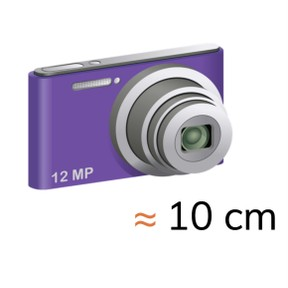Estimating length in centimeters

# Estimating length in centimeters

Students learn to estimate length in centimeters.

No account needed.8,000 schools use Gynzy92,000 teachers use Gynzy1,600,000 students use Gynzy

## General

Students learn to estimate the length of familiar objects in centimeters.

## Common core standard(s)

CCSS.Math.Content.2.MD.A.3

## Learning objective

Students will be able to estimate the length of familiar objects in centimeters.

## Introduction

Have the students discuss in groups. Together, they have to think of five objects that are larger than one centimeter and five objects that are smaller than one centimeter, as fast as they can. As soon as they have written all of the objects, the whole group stands up to show that they are done.

## Instruction

To check that students can estimate the length of objects in centimeters, you can ask the following questions:
- What is about one centimeter?
- How can you estimate the length of an object?

## Quiz

The students first practice with a statement about the length of an object in centimeters. Then they must estimate the length of the object, for which they can choose from several possible answers.

## Closing

You discuss with the students again how it is useful to be able to estimate the length of objects, so that you know about how long something is. You check if the students can estimate length using several objects on the interactive whiteboard. With each object there are possible answers. Next to each object is a section of an an image. The students must state what the correct answer is. Then, drag the image sections to the correct answer box. In this way you complete the puzzle to form a complete image.

## Teaching tips

When students have trouble estimating the length of objects, you can remind them that the width of your thumb is about one centimeter and the distance between your thumb to the tip of your pointer finger is about 10 cm. You can compare real objects to your thumb or hand. This is how you can estimate the length of an object.

## Instruction materials

A ruler.

### The online teaching platform for interactive whiteboards and displays in schools

• Save time building lessons

• Manage the classroom more efficiently

• Increase student engagement